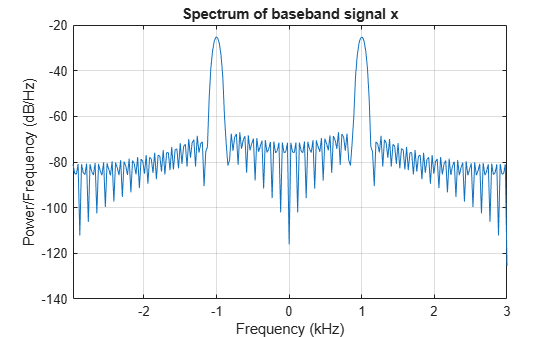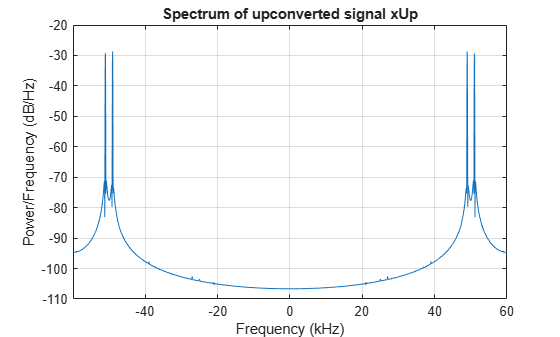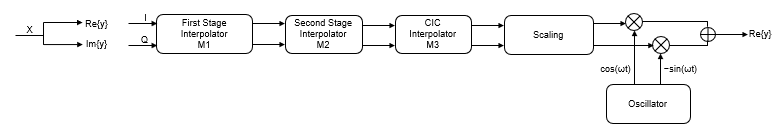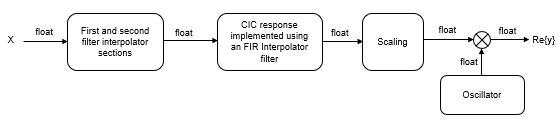Documentation

# dsp.DigitalUpConverter

Interpolate digital signal and translate it from baseband to Intermediate Frequency (IF) band

## Description

The `dsp.DigitalUpConverter` object interpolates a digital signal, and translates it from baseband to Intermediate Frequency (IF) band.

To digitally up convert the input signal:

1. Create the `dsp.DigitalUpConverter` object and set its properties.

2. Call the object with arguments, as if it were a function.

To learn more about how System objects work, see What Are System Objects? (MATLAB).

## Creation

### Syntax

``upConv = dsp.DigitalUpConverter``
``upConv = dsp.DigitalUpConverter(Name,Value)``

### Description

````upConv = dsp.DigitalUpConverter` returns a digital up-converter (DUC) System object™, `upConv`.```

example

````upConv = dsp.DigitalUpConverter(Name,Value)` returns a DUC object, `upConv`, with the specified property `Name` set to the specified value `Value`. You can specify additional name-value pair arguments in any order as (`Name1`,`Value1`,...,`NameN`,`ValueN`).```

## Properties

expand all

Unless otherwise indicated, properties are nontunable, which means you cannot change their values after calling the object. Objects lock when you call them, and the `release` function unlocks them.

If a property is tunable, you can change its value at any time.

For more information on changing property values, see System Design in MATLAB Using System Objects (MATLAB).

Set this property to a positive scalar value. The value of this property multiplied by the total interpolation factor must be greater than or equal to twice the value of the `CenterFrequency` property.

Data Types: `single` | `double`

Interpolation factor, specified as a positive, integer scalar, or to a 1-by-2 or 1-by-3 vector of positive integers.

When you set this property to a scalar the object automatically chooses the interpolation factors for each of the three filtering stages.

When you set this property to a 1-by-2 vector, the object bypasses the first filter stage and sets the interpolation factor of the second and third filtering stages to the values in the first and second vector elements respectively. Both elements of the `InterpolationFactor` vector must be greater than one.

When you set this property to a 1-by-3 vector, the ith element of the vector specifies the interpolation factor for the ith filtering stage. The second and third elements of the `InterpolationFactor` vector must be greater than one and the first element must be `1` or `2`.

Data Types: `double`

Minimum order filter design, specified as `true` or `false`.

When you set this property to `true`, the object designs filters with the minimum order that meets the passband ripple, stopband attenuation, passband frequency, and stopband frequency specifications that you set using the `PassbandRipple`, `StopbandAttenuation`, `Bandwidth`, `StopbandFrequencySource`, and `StopbandFrequency` properties.

When you set this property to `false`, the object designs filters with orders that you specify in the `FirstFilterOrder`, `SecondFilterOrder`, and `NumCICSections` properties. The filter designs meet the passband and stopband frequency specifications that you set using the `Bandwidth`, `StopbandFrequencySource`, and `StopbandFrequency` properties.

Data Types: `logical`

Order of CIC compensation filter stage, specified as a positive, integer scalar.

#### Dependencies

This property applies when you set the `MinimumOrderDesign` property to `false`.

Data Types: `double`

Order of first filter stage, specified as a positive even integer scalar.

#### Dependencies

This property applies when you set the `MinimumOrderDesign` property to `false`. When you set the `InterpolationFactor` property to a 1-by-2 vector, the object ignores the `FirstFilterOrder` property because the first filter stage is bypassed.

Data Types: `double`

Number of sections of CIC interpolator, specified as a positive, integer scalar.

#### Dependencies

This property applies when you set the `MinimumOrderDesign` property to `false`.

Data Types: `double`

Two-sided bandwidth of input signal in Hz, specified as a positive, integer scalar. The object sets the passband frequency of the cascade of filters to one-half of the value that you specify in the `Bandwidth` property.

Data Types: `double`

Source of stopband frequency, specified as `Auto` or `Property`. When you set this property to `Auto`, the object places the cutoff frequency of the cascade filter response at approximately ```Fc = SampleRate/2``` Hz, and computes the stopband frequency as ```Fstop = Fc + TW/2```. TW is the transition bandwidth of the cascade response, computed as `2×(Fc–Fp)`, and the passband frequency, Fp, equals `Bandwidth/2`.

Stopband frequency in Hz., specified as a positive scalar.

#### Dependencies

This property applies when you set the `StopbandFrequencySource` property to `Property`.

Data Types: `double`

Passband ripple of cascade response in dB, specified as a double precision positive scalar. When you set the `MinimumOrderDesign` property to `true`, the object designs the filters so that the cascade response meets the passband ripple that you specify in the `PassbandRipple` property.

#### Dependencies

This property applies when you set the `MinimumOrderDesign` property to `true`.

Data Types: `double`

Stopband attenuation of cascade response in dB, specified as a double precision positive scalar. When you set the `MinimumOrderDesign` property to `true`, the object designs the filters so that the cascade response meets the stopband attenuation that you specify in the `StopbandAttenuation` property.

#### Dependencies

This property applies when you set the `MinimumOrderDesign` property to `true`.

Data Types: `double`

Type of oscillator, specified as either `Sine wave` or `NCO`. When you set this property to `Sine wave`, the object frequency up converts the output of the interpolation filter cascade using a complex exponential signal obtained from samples of a sinusoidal trigonometric function. When you set this property to `NCO` the object performs frequency up conversion with a complex exponential obtained using a numerically controlled oscillator (NCO).

Center frequency of output signal in Hz, specified as a double precision positive scalar. The value of this property must be less than or equal to half the product of the `SampleRate` property times the total interpolation factor. The object up converts the input signal so that the output spectrum centers at the frequency you specify in the `CenterFrequency` property.

Data Types: `double`

### NCO Properties

Number of NCO accumulator bits, specified as an integer scalar in the range `[1 128]`. See `dsp.NCO`.

#### Dependencies

This property applies when you set the `Oscillator` property to `NCO`.

Data Types: `double`

Number of NCO quantized accumulator bits, specified as an integer scalar in the range`[1 128]`. The value you specify for this property must be less than the value you specify in the `NumAccumulatorBits` property. See `dsp.NCO`.

#### Dependencies

This property applies when you set the `Oscillator` property to `NCO`.

Data Types: `double`

Dither control for NCO, specified as `true` or `false`. When you set this property to `true`, the object uses the number of dither bits specified in the `NumDitherBits` property when applying dither to the NCO signal. See `dsp.NCO`.

#### Dependencies

This property applies when you set the `Oscillator` property to `NCO`.

Data Types: `logical`

Number of NCO dither bits, specified as a positive integer scalar smaller than the number of accumulator bits that you specify in the `NumAccumulatorBits` property. See `dsp.NCO`.

#### Dependencies

This property applies when you set the `Oscillator` property to `NCO` and the `Dither` property to `true`.

Data Types: `double`

### Fixed-Point Properties

Data type at the output of the first (if it has not been bypassed), second, and third filter stages, specified as one of `Same as input` | `Custom`. The object casts the data at the output of each filter stage according to the value you set in this property. For the CIC stage, the casting is done after the signal has been scaled by the normalization factor.

Fixed-point data type of output of each filter stage, specified as a scaled `numerictype` object with a Signedness of `Auto`.

#### Dependencies

This property applies when you set the `FiltersOutputDataType` property to `Custom`.

Data type of output, specified as `Same as input` or `Custom`.

Fixed-point data type of output, specified as a scaled `numerictype` object with a Signedness of `Auto`.

#### Dependencies

This property applies when you set the `OutputDataType` property to `Custom`.

## Usage

For versions earlier than R2016b, use the `step` function to run the System object algorithm. The arguments to `step` are the object you created, followed by the arguments shown in this section.

For example, `y = step(obj,x)` and `y = obj(x)` perform equivalent operations.

### Syntax

``y = upConv(x)``

### Description

example

````y = upConv(x)` takes a real or complex input column vector `x` and outputs an up sampled and frequency up converted signal `y`.```

### Input Arguments

expand all

Data input, specified as a column vector. The length of input `x` must be a multiple of the decimation factor. When the data type of `x` is `double` or `single` precision, the data type of `y` is the same as that of `x`. When the data type of `x` is of a fixed-point type, the data type of `y` is defined by the `OutputDataType` property.

Data Types: `single` | `double` | `int8` | `int16` | `int32` | `int64` | `fi`
Complex Number Support: Yes

### Output Arguments

expand all

Up converted and up sampled signal, returned as a column vector. The length of `y` is equal to the length of `x` divided by the `InterpolationFactor`. When the data type of `x` is `double` or `single` precision, the data type of `y` is the same as that of `x`. When the data type of `x` is of a fixed point type, the data type of `y` is defined by the `OutputDataType` property.

Data Types: `single` | `double` | `int8` | `int16` | `int32` | `int64` | `fi`
Complex Number Support: Yes

## Object Functions

To use an object function, specify the System object as the first input argument. For example, to release system resources of a System object named `obj`, use this syntax:

`release(obj)`

expand all

 `getInterpolationFactors` Get interpolation factors of each filter stage of a digital up converter `getFilterOrders` Get orders of digital down converter or digital up converter filter cascade `getFilters` Get handles to digital down converter or digital up converter filter cascade objects `fvtool` Visualize frequency response of digital down converter or digital up converter filter cascade `groupDelay` Group delay of digital down converter or digital up converter filter cascade `visualizeFilterStages` Display response of digital down converter or digital up converter filter cascade `generatehdl` Generate HDL code for quantized DSP filter (requires Filter Design HDL Coder)
 `step` Run System object algorithm `release` Release resources and allow changes to System object property values and input characteristics `reset` Reset internal states of System object

## Examples

### Upconvert a Sine Wave Signal

Create a digital up converter object that upsamples a 1 KHz sinusoidal signal by a factor of 20, and up converts it to 50 KHz.

Note: This example runs only in R2016b or later. If you are using an earlier release, replace each call to the function with the equivalent `step` syntax. For example, myObject(x) becomes step(myObject,x).

Initialization

Create a sine wave generator to obtain the 1 KHz sinusoidal signal with a sample rate of 6 KHz.

``` Fs = 6e3; % Sample rate sine = dsp.SineWave('Frequency',1000,'SampleRate', Fs,'SamplesPerFrame',1024); x = sine(); % generate signal```

Create a DUC object. Use minimum order filter designs and set the passband ripple to 0.2 dB and the stopband attenuation to 55 dB. Set the double sided signal bandwidth to 2 KHz.

```upConv = dsp.DigitalUpConverter(... 'InterpolationFactor', 20,... 'SampleRate', Fs,... 'Bandwidth', 2e3,... 'StopbandAttenuation', 55,... 'PassbandRipple',0.2,... 'CenterFrequency',50e3);```

Create a spectrum estimator to visualize the signal spectrum before and after up converting.

```window = hamming(floor(length(x)/10)); figure; pwelch(x,window,[],[],Fs,'centered') title('Spectrum of baseband signal x')```Upconvert the Signal and Visualize the Spectrum

``` xUp = upConv(x); % up convert window = hamming(floor(length(xUp)/10)); figure; pwelch(xUp,window,[],[],20*Fs,'centered') title('Spectrum of up converted signal xUp')```Visualize the response of the interpolation filters

` visualizeFilterStages(upConv)`## Algorithms

The object up samples the input signal using a cascade of three interpolation filters. This object frequency-upconverts the up-sampled signal by multiplying it with a complex exponential with center frequency equal to the value in the `CenterFrequency` property. In this case, the filter cascade consists of a first FIR interpolation stage, a second stage for CIC compensation, and a CIC interpolator. The block diagram shows the architecture of the digital up converter.The scaling section normalizes the CIC gain and the oscillator power. It may also contain a correction factor to achieve the desired ripple specification. Depending on the setting of the `InterpolationFactor` property, you may be able to bypass the first filter stage. When the input data type is floating-point, the object implements an N-section CIC interpolation filter as an FIR filter with a response that corresponds to a cascade of N boxcar filters. The CIC filter is emulated with an FIR filter so that you can run simulations with floating-point data. When the input data is fixed-point type, the object implements a true CIC filter with actual comb and integrator sections.

The following diagram represents the DUC arithmetic with single or double-precision, floating-point inputs.For details of fixed-point operation, see Fixed Point.

Watch now